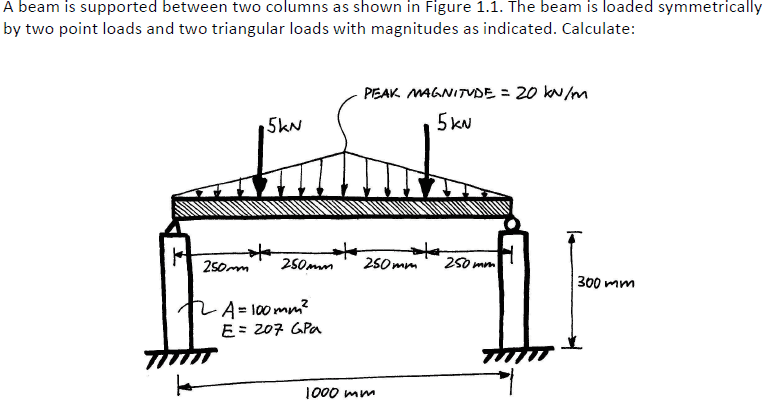# Civil Engineering - beam question

• jumbo123

## Homework Statement(a) The total load applied to the beam and its direction (in kN)
(b) The stress (σ) in each column given that Their cross-sections are solid square sections 10 x 10 mm
(in MPa)
(c) The strain in each column if the Young’s Modulus (E) of the steel is 207 GPa.
(d) The internal shear (V), axial (N), and bending moment (M) in the beam at its mid-length.
(e) The distance (in mm) that the left hand end of the beam moves down under the given loading.

## The Attempt at a Solution

I think that a is 20kN. B is 100MPa. C is 4.83x10^-4.

I don@t know what to do from here. Any help is appreciated.

For Part d, you should draw a FBD of the beam showing the loads and the reactions at the supports.